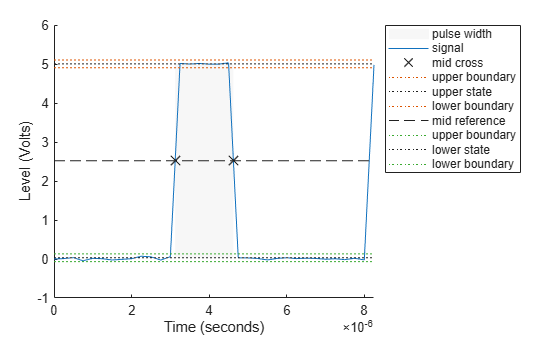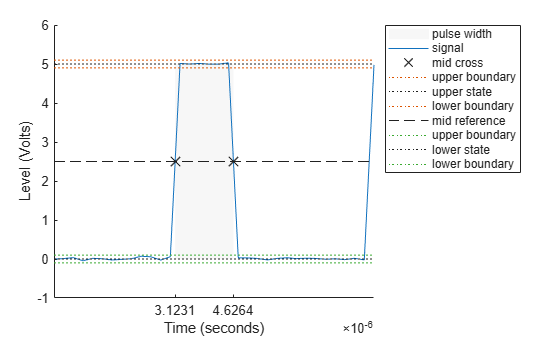Documentation

# pulsewidth

Bilevel waveform pulse width

## Syntax

```W = pulsewidth(X) W = pulsewidth(X,FS) W = pulsewidth(X,T) [W,INITCROSS] = pulsewidth(...) [W,INITCROSS,FINALCROSS] = pulsewidth(...) [W,INITCROSS,FINALCROSS,MIDLEV] = pulsewidth(...) W = pulsewidth(...,Name,Value) pulsewidth(...) ```

## Description

`W = pulsewidth(X)` returns a vector, `W`, containing the time differences between the mid-reference level instants of the initial and final transitions of each positive-polarity pulse in the bilevel waveform, `X`. To determine the transitions, `pulsewidth` estimates the low- and high-state levels of `X` by a histogram method. `pulsewidth` identifies all regions that cross the upper-state boundary of the low state and the lower-state boundary of the high state. The low-state and high-state boundaries are expressed as the state level plus or minus a multiple of the difference between the state levels. See State-Level Tolerances. Because `pulsewidth` uses interpolation to determine the mid-reference level instants, `W` may contain values that do not correspond to sampling instants of the bilevel waveform, `X`.

`W = pulsewidth(X,FS)` specifies the sample rate, `FS`, in hertz as a positive scalar. The first sample in the waveform corresponds to t=0. Because `pulsewidth` uses interpolation to determine the mid-reference level instants, `W` may contain values that do not correspond to sampling instants of the bilevel waveform, `X`.

`W = pulsewidth(X,T)` specifies the sample instants, `T`, as a vector with the same number of elements as `X`. Because `pulsewidth` uses interpolation to determine the mid-reference level instants, `W` may contain values that do not correspond to sampling instants of the bilevel waveform, `X`.

```[W,INITCROSS] = pulsewidth(...)``` returns a column vector, `INITCROSS`, whose elements correspond to the mid-reference level instants of the initial transition of each pulse.

```[W,INITCROSS,FINALCROSS] = pulsewidth(...)``` returns a column vector, `FINALCROSS`, whose elements correspond to the mid-reference level instants of the final transition of each pulse.

```[W,INITCROSS,FINALCROSS,MIDLEV] = pulsewidth(...)``` returns the waveform value, `MIDLEV`, which corresponds to the mid-reference level.

`W = pulsewidth(...,Name,Value)` returns the pulse widths with additional options specified by one or more `Name,Value` pair arguments.

`pulsewidth(...)` plots the signal and darkens the regions of each pulse where pulse width is computed. It marks the location of the mid crossings, and their associated reference level. The state levels and their associated lower and upper boundaries (adjustable by the `Name,Value` pair with name `'Tolerance'`) are also plotted.

## Input Arguments

 `X` Bilevel waveform. `X` is a real-valued row or column vector. `FS` Sample rate in hertz. `T` Vector of sample instants. The length of `T` must equal the length of the bilevel waveform, `X`.

### Name-Value Pair Arguments

 `'MidPercentReferenceLevel'` Mid-reference level as percentage of the waveform amplitude. See Mid-Reference Level. Default: `50` `'Polarity'` Pulse polarity. Specify the polarity as `'positive'` or `'negative'`. If you specify `'positive'`, `pulsewidth` looks for pulses with positive-going (positive polarity) initial transitions. If you specify `'negative'`, `pulsewidth` looks for pulses with negative-going (negative polarity) initial transitions. See Pulse Polarity. Default: `'positive'` `'StateLevels'` Low- and high-state levels. `StateLevels` is a 1-by-2 real-valued vector. The first element is the low-state level. The second element is the high-state level. If you do not specify low- and high-state levels, `pulsewidth` estimates the state levels from the input waveform using the histogram method. `'Tolerance'` Tolerance levels (lower and upper state boundaries) expressed as a percentage. See State-Level Tolerances. Default: `2`

## Output Arguments

 `W` Pulse widths in seconds. The pulse width is the time difference between the initial and final transitions of a pulse. The times of the initial and final transitions are referred to as transition occurrence instants in . `INITCROSS` Mid-reference level instants of the initial transition `FINALCROSS` Mid-reference level instants of the final transition `MIDLEV` Waveform value corresponding to the mid-reference level

## Examples

collapse all

Compute the pulse width of a bilevel waveform sampled at 4 MHz.

```load('pulseex.mat','x','t') w = pulsewidth(x,t)```
```w = 1.5016e-06 ```

Plot the waveform and annotate the pulse width.

`pulsewidth(x,t);`Compute the initial and final transition occurrences for a bilevel waveform sampled at 4 MHz.

```load('pulseex.mat', 'x', 't'); fs = 4e6; [w,initcross,finalcross] = pulsewidth(x,fs);```

Plot the result, annotated with the transition occurrences.

```pulsewidth(x,fs); ax = gca; ax.XTick = [initcross finalcross];```Specify the state levels for the bilevel waveform instead of estimating the levels from the data. Use the `'StateLevels'` name-value pair to enter the low-state level as 0 and the high-state level as 5.

```load('pulseex.mat', 'x', 't') fs = 4e6; [w,initcross,finalcross] = pulsewidth(x,fs,'StateLevels',[0 5]);```

Plot the result annotated with the transition occurrences.

```pulsewidth(x,fs,'StateLevels',[0 5]); ax = gca; ax.XTick = [initcross finalcross];```collapse all

### Mid-Reference Level

The mid-reference level in a bilevel waveform with low-state level, S1, and high- state level, S2, is

`${S}_{1}+\frac{1}{2}\left({S}_{2}-{S}_{1}\right)$`

### Mid-Reference Level Instant

Let y50% denote the mid-reference level.

Let t50%- and t50%+ denote the two consecutive sampling instants corresponding to the waveform values nearest in value to y50%.

Let y50%- and y50%+ denote the waveform values at t50%- and t50%+.

The mid-reference level instant is

`${t}_{50%}={t}_{50%}+\left(\frac{{t}_{50{%}_{+}}-{t}_{50{%}_{-}}}{{y}_{50{%}_{+}}-{y}_{50{%}_{-}}}\right)\left({y}_{50{%}_{+}}-{y}_{50{%}_{-}}\right)$`

### Pulse Polarity

If the pulse has a positive-going initial transition, the pulse has positive polarity. The following figure shows a positive-polarity pulse.Equivalently, a positive-polarity (positive-going) pulse has a terminating state more positive than the originating state.

If the pulse has a negative-going initial transition, the pulse has negative polarity. The following figure shows a negative-polarity pulse.Equivalently, a negative-polarity (negative-going) pulse has a originating state more positive than the terminating state.

### State-Level Tolerances

Each state level can have associated lower- and upper-state boundaries. These state boundaries are defined as the state level plus or minus a scalar multiple of the difference between the high state and the low state. To provide a useful tolerance region, the scalar is typically a small number such as 2/100 or 3/100. In general, theregion for the low state is defined as

``

whereis the low-state level andis the high-state level. Replace the first term in the equation withto obtain thetolerance region for the high state.

The following figure illustrates lower and upper 2% state boundaries (tolerance regions) for a positive-polarity bilevel waveform. The red dashed lines indicate the estimated state levels.## References

 IEEE® Standard on Transitions, Pulses, and Related Waveforms, IEEE Standard 181, 2003.